# Vibration

Classical mechanics
History · Timeline

Vibration refers to mechanical oscillations about an equilibrium point. The oscillations may be periodic, such as the motion of a pendulum, or random, such as the movement of a tire on a gravel road. Vibrations are closely related to sound, which takes the form of "pressure waves." These waves are generated by vibrating structures, such as vocal cords, musical instruments, and loudspeakers. The same waves can induce the vibration of other structures, such as the ear drum.

Many types of vibrations are deemed undesirable, as they waste energy and create unwanted sounds, which most people call noise. For example, the vibrational motions of engines, electric motors, or any mechanical device in operation are typically unwanted. Such vibrations can be caused by imbalances in the rotating parts, uneven friction, the meshing of gear teeth, and so forth. Careful designs are needed to minimize unwanted vibrations.

## Types of vibration

Free vibration occurs when a mechanical system is set off with an initial input and then allowed to vibrate freely. Examples of this type of vibration are pulling a child back on a swing and then letting go or hitting a tuning fork and letting it ring. The mechanical system will then vibrate at one or more of its "natural frequencies" and damp down to zero.

Forced vibration is when an alternating force or motion is applied to a mechanical system. Examples of this type of vibration include a shaking washing machining due to an imbalance, transportation vibration (caused by truck engine, springs, road, and so on), or the vibration of a building during an earthquake. In forced vibration the frequency of the vibration is the frequency of the force or motion applied, with order of magnitude being dependent on the actual mechanical system.

## Vibration testing

Vibration testing is accomplished by introducing a forcing function into a structure, usually with some type of shaker. Generally, one or more points on the structure are kept at a specified vibration level. Two typical types of vibration tests performed are random and sine test. Sine tests are performed to survey the structural response of the device under test (DUT). A random test is generally conducted to more closely replicate a real world environment.

Most vibration testing is conducted in the vertical axis. Some may be conducted horizontally, in multiple axes, or rotationally.

## Vibration analysis

The fundamentals of vibration analysis can be understood by studying the simple mass-spring-damper model. Indeed, even a complex structure such as an automobile body can be modeled as a "summation" of simple mass-spring-damper models. The mass-spring-damper model is an example of a simple harmonic oscillator. The mathematics used to describe its behavior is identical to other simple harmonic oscillators such as the RLC circuit.

Note: In this article the step by step mathematical derivations will not be included, but will focus on the major equations and concepts in vibration analysis. Please refer to the references at the end of the article for detailed derivations.

### Free vibration without damping

To start the investigation of the mass-spring-damper we will assume the damping is negligible and that there is no external force applied to the mass (that is, free vibration).

The force applied to the mass by the spring is proportional to the amount the spring is stretched "x" (we will assume the spring is already compressed due to the weight of the mass). The proportionality constant, k, is the stiffness of the spring and has units of force/distance (e.g. lbf/in or N/m)$F_s=- k x \!$

The force generated by the mass is proportional to the acceleration of the mass as given by Newton’s second law of motion.$\Sigma\ F = ma = m \ddot{x} = m \frac{d^2x}{dt^2}$

The sum of the forces on the mass then generates this ordinary differential equation: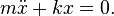$m \ddot{x} + k x = 0.$

If we assume that we start the system to vibrate by stretching the spring by the distance of A and letting go, the solution to the above equation that describes the motion of mass is: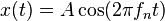$x(t) = A \cos (2 \pi f_n t) \!$

This solution says that it will oscillate with simple harmonic motion that has an amplitude of A and a frequency of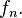$f_n.$ The number$f_n$ is one of the most important quantities in vibration analysis and is called the undamped natural frequency. For the simple mass-spring system,$f_n$ is defined as: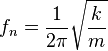$f_n = {1\over {2 \pi}} \sqrt{k \over m} \!$

Note: Angular frequency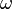$\omega$ (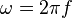$\omega=2 \pi f$) with the units of radians per second is often used in equations because it simplifies the equations, but is normally converted to “standard” frequency (units of Hz or equivalently cycles per second) when stating the frequency of a system.

If you know the mass and stiffness of the system you can determine the frequency at which the system will vibrate once it is set in motion by an initial disturbance using the above stated formula. Every vibrating system has one or more natural frequencies that it will vibrate at once it is disturbed. This simple relation can be used to understand in general what will happen to a more complex system once we add mass or stiffness. For example, the above formula explains why when a car or truck is fully loaded the suspension will feel “softer” than unloaded because the mass has increased and therefore reduced the natural frequency of the system.

#### What causes the system to vibrate under no force?

These formulas describe the resulting motion, but they do not explain why the system oscillates. The reason for the oscillation is due to the conservation of energy. In the above example we have extended the spring by a value of A and therefore have stored potential energy (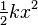$\tfrac {1}{2} k x^2$) in the spring. Once we let go of the spring, the spring tries to return to its un-stretched state and in the process accelerates the mass. At the point where the spring has reached its un-stretched state it no longer has energy stored, but the mass has reached its maximum speed and hence all the energy has been transformed into kinetic energy (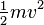$\tfrac {1}{2} m v^2$). The mass then begins to decelerate because it is now compressing the spring and in the process transferring the kinetic energy back to its potential. This transferring back and forth of the kinetic energy in the mass and the potential energy in the spring causes the mass to oscillate.

In this simple model, the mass will continue to oscillate forever at the same magnitude, but in a real system there is always something called damping that dissipates the energy and therefore the system eventually bringing it to rest.

### Free vibration with damping

We now add a "viscous" damper to the model that outputs a force that is proportional to the velocity of the mass. The damping is called viscous because it models the effects of an object within a fluid. The proportionality constant c is called the damping coefficient and has units of Force over velocity (lbf s/ in or N s/m).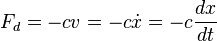$F_d = - c v = - c \dot{x} = - c \frac{dx}{dt} \!$

By summing the forces on the mass, it yields the following ordinary differential equation:$m \ddot{x} + { c } \dot{x} + {k } x = 0.$

The solution to this equation depends on the amount of damping. If the damping is small enough the system will still vibrate, but eventually, over time, will stop vibrating. This case is called underdamping—this case is of most interest in vibration analysis. If we increase the damping just to the point where the system no longer oscillates we reach the point of critical damping (if the damping is increased past critical damping the system is called overdamped). The value that the damping coefficient needs to reach for critical damping in the mass spring damper model is: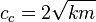$c_c= 2 \sqrt{k m}$

To characterize the amount of damping in a system a ratio called the damping ratio (also known as damping factor and % critical damping) is used. This damping ratio is just a ratio of the actual damping over the amount of damping required to reach critical damping. The formula for the damping ratio ($\zeta$) of the mass spring damper model is: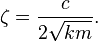$\zeta = { c \over 2 \sqrt{k m} }.$

For example, metal structures (for example, airplane fuselage, engine crankshaft) will have damping factors less than 0.05 while automotive suspensions in the range of 0.2-0.3.

The solution to the underdamped system for the mass spring damper model is the following:$x(t)=X e^{-\zeta \omega_n t} \cos({\sqrt{1-\zeta^2} \omega_n t - \phi}) , \ \ \omega_n= 2\pi f_n$

The value of X, the initial magnitude, and$\phi$, the phase shift, are determined by the amount the spring is stretched. The formulas for these values can be found in the references.

The major points to note from the solution are the exponential term and the cosine function. The exponential term defines how quickly the system “damps” down—the larger the damping ratio, the quicker it damps to zero. The cosine function is the oscillating portion of the solution, but the frequency of the oscillations is different from the undamped case.

The frequency in this case is called the "damped natural frequency,"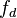$f_d$, and is related to the undamped natural frequency by the following formula: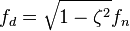$f_d= \sqrt{1-\zeta^2} f_n$

The damped natural frequency is less than the undamped natural frequency, but for many practical cases the damping ratio is relatively small and hence the difference is negligible. Therefore the damped and undamped description are often dropped when stating the natural frequency (for example, with 0.1 damping ratio, the damped natural frequency is only 1 percent less than the undamped).

The plots to the side present how 0.1 and 0.3 damping ratios effect how the system will “ring” down over time. What is often done in practice is to experimentally measure the free vibration after an impact (for example by a hammer) and then determine the natural frequency of the system by measuring the rate of oscillation as well as the damping ratio by measuring the rate of decay. The natural frequency and damping ratio are not only important in free vibration, but also characterize how a system will behave under forced vibration.

### Forced vibration with damping

In this section we will look at the behavior of the spring mass damper model when we add a harmonic force in the form below. A force of this type could, for example, be generated by a rotating imbalance.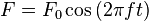$F= F_0 \cos {(2 \pi f t)} \!$

If we again sum the forces on the mass we get the following ordinary differential equation:$m \ddot{x} + { c } \dot{x} + {k } x = F_0 \cos {(2 \pi f t)}$

The steady state solution of this problem can be written as: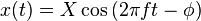$x(t)= X \cos {(2 \pi f t -\phi)} \!$

The result states that the mass will oscillate at the same frequency, f, of the applied force, but with a phase shift$\phi$.

The amplitude of the vibration “X” is defined by the following formula.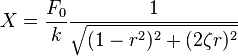$X= {F_0 \over k} {1 \over \sqrt{(1-r^2)^2 + (2 \zeta r)^2}}$

Where “r” is defined as the ratio of the harmonic force frequency over the undamped natural frequency of the mass-spring-damper model.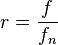$r=\frac{f}{f_n}$

The phase shift,$\phi$, is defined by following formula.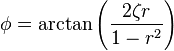$\phi= \arctan {\left (\frac{2 \zeta r}{1-r^2} \right)}$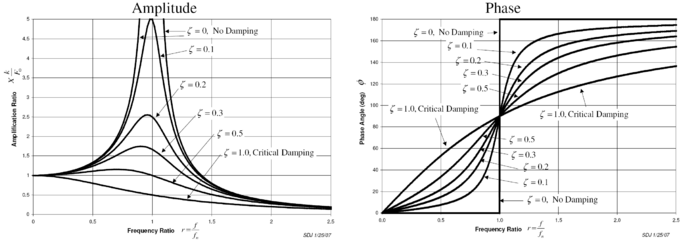The plot of these functions, called "the frequency response of the system," presents one of the most important features in forced vibration. In a lightly damped system when the forcing frequency nears the natural frequency (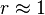$r \approx 1$) the amplitude of the vibration can get extremely high. This phenomenon is called resonance (subsequently the natural frequency of a system is often referred to as the resonant frequency). In rotor bearing systems any rotational speed that excites a resonant frequency is referred to as a critical speed.

If resonance occurs in a mechanical system it can be very harmful—leading to eventual failure of the system. Consequently, one of the major reasons for vibration analysis is to predict when this type of resonance may occur and then to determine what steps to take to prevent it from occurring. As the amplitude plot shows, adding damping can significantly reduce the magnitude of the vibration. Also, the magnitude can be reduced if the natural frequency can be shifted away from the forcing frequency by changing the stiffness or mass of the system. If the system cannot be changed, perhaps the forcing frequency can be shifted (for example, changing the speed of the machine generating the force).

The following are some other points in regards to the forced vibration shown in the frequency response plots.

• At a given frequency ratio, the amplitude of the vibration, X, is directly proportional to the amplitude of the force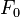$F_0$ (for example, if one doubles the force, the vibration doubles)
• With little or no damping, the vibration is in phase with the forcing frequency when the frequency ratio r < 1 and 180 degrees out of phase when the frequency ratio r >1
• When r<<1 the amplitude is just the deflection of the spring under the static force$F_0$. This deflection is called the static deflection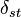$\delta_{st}$. Hence, when r<<1 the effects of the damper and the mass are minimal.
• When r>>1 the amplitude of the vibration is actually less than the static deflection$\delta_{st}$. In this region the force generated by the mass (F=ma) is dominating because the acceleration seen by the mass increases with the frequency. Since the deflection seen in the spring, X, is reduced in this region, the force transmitted by the spring (F=kx) to the base is reduced. Therefore the mass-spring-damper system is isolating the harmonic force from the mounting base—referred to as vibration isolation. Interestingly, more damping actually reduces the effects of vibration isolation when r>>1 because the damping force (F=cv) is also transmitted to the base.

#### What causes resonance?

Resonance is simple to understand if you view the spring and mass as energy storage elements—with the mass storing kinetic energy and the spring storing potential energy. As discussed earlier, when the mass and spring have no force acting on them they transfer energy back and forth at a rate equal to the natural frequency. In other words, if energy is to be efficiently pumped into both the mass and spring the energy source needs to feed the energy in at a rate equal to the natural frequency. Applying a force to the mass and spring is similar to pushing a child on swing, one needs to push at the correct moment if you want the swing to get higher and higher. As in the case of the swing, the force applied does not necessarily have to be high to get large motions; the pushes just need to keep adding energy into the system.

The damper, instead of storing energy, dissipates energy. Since the damping force is proportional to the velocity, the more the motion the more the damper dissipates the energy. Therefore a point will come when the energy dissipated by the damper will equal the energy being fed in by the force. At this point, the system has reached its maximum amplitude and will continue to vibrate at this level as long as the force applied stays the same. If no damping exists, there is nothing to dissipate the energy and therefore theoretically the motion will continue to grow on into infinity.

#### Applying "complex" forces to the mass-spring-damper model

In a previous section only a simple harmonic force was applied to the model, but this can be extended considerably using two powerful mathematical tools. The first is the Fourier transform that takes a signal as a function of time (time domain) and breaks it down into its harmonic components as a function of frequency (frequency domain). For example, let us apply a force to the mass-spring-damper model that repeats the following cycle—a force equal to 1 newton for 0.5 second and then no force for 0.5 second. This type of force has the shape of a 1 Hz square wave.

The Fourier transform of the square wave generates a frequency spectrum that presents the magnitude of the harmonics that make up the square wave (the phase is also generated, but is typically of less concern and therefore is often not plotted). The Fourier transform can also be used to analyze non-periodic functions such as transients (for example, impulses) and random functions. With the advent of the modern computer the Fourier transform is almost always computed using the Fast Fourier Transform (FFT) computer algorithm in combination with a window function.

In the case of our square wave force, the first component is actually a constant force of 0.5 newton and is represented by a value at "0" Hz in the frequency spectrum. The next component is a 1 Hz sine wave with an amplitude of 0.64. This is shown by the line at 1 Hz. The remaining components are at odd frequencies and it takes an infinite amount of sine waves to generate the perfect square wave. Hence, the Fourier transform allows you to interpret the force as a sum of sinusoidal forces being applied instead of a more "complex" force (for example, a square wave).

In the previous section, the vibration solution was given for a single harmonic force, but the Fourier transform will in general give multiple harmonic forces. The second mathematical tool, "the principle of superposition", allows you to sum the solutions from multiple forces if the system is linear. In the case of the spring-mass-damper model, the system is linear if the spring force is proportional to the displacement and the damping is proportional to the velocity over the range of motion of interest. Hence, the solution to the problem with a square wave is summing the predicted vibration from each one of the harmonic forces found in the frequency spectrum of the square wave.

#### Frequency response model

We can view the solution of a vibration problem as an input/output relation—where the force is the input and the output is the vibration. If one represents the force and vibration in the frequency domain (magnitude and phase) we can write the following relation: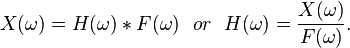$X(\omega)=H(\omega)* F(\omega) \ \ or \ \ H(\omega)= {X(\omega) \over F(\omega)}.$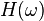$H(\omega)$ is called the frequency response function (also referred to as the transfer function, but not technically as accurate) and has both a magnitude and phase component (if represented as a complex number, a real and imaginary component). The magnitude of the frequency response function (FRF) was presented earlier for the mass-spring-damper system.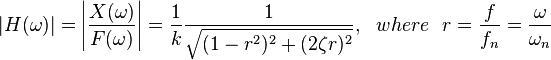$|H(\omega)|=\left |{X(\omega) \over F(\omega)} \right|= {1 \over k} {1 \over \sqrt{(1-r^2)^2 + (2 \zeta r)^2}}, \ \ where\ \ r=\frac{f}{f_n}=\frac{\omega}{\omega_n}$

The phase of the FRF was also presented earlier as:$\angle H(\omega)= \arctan {\left (\frac{2 \zeta r}{1-r^2} \right)}.$

For example, let us calculate the FRF for a mass-spring-damper system with a mass of 1 kg, spring stiffness of 1.93 N/mm and a damping ratio of 0.1. The values of the spring and mass give a natural frequency of 7 Hz for this specific system. If we apply the 1 Hz square wave from earlier one can calculate the predicted vibration of the mass. The figure illustrates the resulting vibration. It happens in this example that the fourth harmonic of the square wave falls at 7 Hz. The frequency response of the mass-spring-damper therefore outputs a high 7 Hz vibration even though the input force had a relatively low 7 Hz harmonic. This example highlights that the resulting vibration is dependent on both the forcing function and the system that the force is applied to.

The figure also shows the time domain representation of the resulting vibration. This is done by performing an inverse Fourier Transform that converts frequency domain data to time domain. In practice, this is rarely done because the frequency spectrum provides all the necessary information.

The frequency response function (FRF) does not necessarily have to be calculated from the knowledge of the mass, damping, and stiffness of the system, but can be measured experimentally. For example, if you apply a known force and sweep the frequency and then measure the resulting vibration you can calculate the frequency response function and then characterize the system. This technique is used in the field of experimental modal analysis to determine the vibration characteristics of a structure.

## Multiple degrees of freedom systems and mode shapes

The simple mass-spring damper model is the foundation of vibration analysis, but what about more complex systems? The mass-spring-damper model described above is called a single degree of freedom (DOF) model since we have assumed the mass only moves up and down. In the case of more complex systems we need to discretize the system into more masses and allow them to move in more than one direction—adding degrees of freedom. The major concepts of multiple degrees of freedom (MDOF) can be understood by looking at just a 2 degree of freedom model as shown in the figure.

The equations of motion of the 2DOF system are found to be:$m_1 \ddot{x_1} + { (c_1+c_2) } \dot{x_1} - { c_2 } \dot{x_2}+ { (k_1+k_2) } x_1 -{ k_2 } x_2= f_1$$m_2 \ddot{x_2} - { c_2 } \dot{x_1}+ { (c_2+c_3) } \dot{x_2} - { c_3 } \dot{x_3} - { k_2 } x_1+ { (k_2+k_3) } x_2 -{ k_3 } x_3= f_2 \!$

One can rewrite this in matrix format: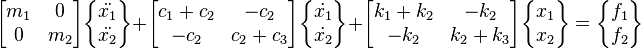$\begin{bmatrix}m_1 & 0\\ 0 & m_2\end{bmatrix}\begin{Bmatrix}\ddot{x_1}\\ \ddot{x_2}\end{Bmatrix}+\begin{bmatrix}c_1+c_2 & -c_2\\ -c_2 & c_2+c_3\end{bmatrix}\begin{Bmatrix}\dot{x_1}\\ \dot{x_2}\end{Bmatrix}+\begin{bmatrix}k_1+k_2 & -k_2\\ -k_2 & k_2+k_3\end{bmatrix}\begin{Bmatrix} x_1\\ x_2\end{Bmatrix}=\begin{Bmatrix} f_1\\ f_2\end{Bmatrix}$

A more compact form of this matrix equation can be written as:$\begin{bmatrix}M\end{bmatrix}\begin{Bmatrix}\ddot{x}\end{Bmatrix}+\begin{bmatrix}C\end{bmatrix}\begin{Bmatrix}\dot{x}\end{Bmatrix}+\begin{bmatrix}K\end{bmatrix}\begin{Bmatrix} x\end{Bmatrix}=\begin{Bmatrix} f \end{Bmatrix} \!$

where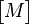$\begin{bmatrix}M\end{bmatrix}$,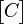$\begin{bmatrix}C\end{bmatrix}$, and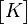$\begin{bmatrix}K\end{bmatrix}$ are symmetric matrices referred respectively as the mass, damping, and stiffness matrices. The matrices are NxN square matrices where N is the number of degrees of freedom of the system.

In the following analysis we will consider the case where there is no damping and no applied forces (that is, free vibration). The solution of a viscously damped system is somewhat more complicated and is shown in Maia.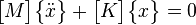$\begin{bmatrix}M\end{bmatrix}\begin{Bmatrix}\ddot{x}\end{Bmatrix}+\begin{bmatrix}K\end{bmatrix}\begin{Bmatrix} x\end{Bmatrix}=0$

This differential equation can be solved by assuming the following type of solution: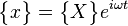$\begin{Bmatrix} x\end{Bmatrix}=\begin{Bmatrix} X\end{Bmatrix}e^{i\omega t}$

Note: Using the exponential solution of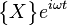$\begin{Bmatrix} X\end{Bmatrix}e^{i\omega t}$ is a mathematical trick used to solve linear differential equations. If we use Euler's formula and take only the real part of the solution it is the same cosine solution for the 1 DOF system. The exponential solution is only used because it easier to manipulate mathematically.

The equation then becomes: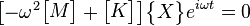$\begin{bmatrix}-\omega^2\begin{bmatrix}M\end{bmatrix}+\begin{bmatrix}K\end{bmatrix}\end{bmatrix}\begin{Bmatrix}X\end{Bmatrix}e^{i\omega t}=0$

Since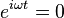$e^{i\omega t}=0$ cannot equal zero the equation reduces to the following.$\begin{bmatrix}\begin{bmatrix}K\end{bmatrix}-\omega^2\begin{bmatrix}M\end{bmatrix}\end{bmatrix}\begin{Bmatrix}X\end{Bmatrix}=0$

### Eigenvalue problem

This is referred to an eigenvalue problem in mathematics and can be put in the standard format by pre-multiplying the equation by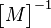$\begin{bmatrix}M\end{bmatrix}^{-1}$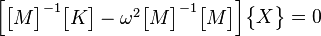$\begin{bmatrix}\begin{bmatrix}M\end{bmatrix}^{-1}\begin{bmatrix}K\end{bmatrix}-\omega^2\begin{bmatrix}M\end{bmatrix}^{-1}\begin{bmatrix}M\end{bmatrix}\end{bmatrix}\begin{Bmatrix}X\end{Bmatrix}=0$

and if we let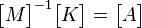$\begin{bmatrix}M\end{bmatrix}^{-1}\begin{bmatrix}K\end{bmatrix}=\begin{bmatrix}A\end{bmatrix}$ and$\lambda=\omega^2 \,$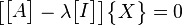$\begin{bmatrix}\begin{bmatrix}A\end{bmatrix}-\lambda\begin{bmatrix}I\end{bmatrix}\end{bmatrix}\begin{Bmatrix}X\end{Bmatrix}=0$

The solution to the problem results in N eigenvalues (ie.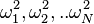$\omega_1^2,\omega_2^2,..\omega_N^2$), where N corresponds to the number of degrees of freedom. The eigenvalues provide the natural frequencies of the system. When these eigenvalues are substituted back into the original set of equations, the values of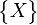$\begin{Bmatrix}X\end{Bmatrix}$ that correspond to each eigenvalue are called the eigenvectors. These eigenvectors represent the mode shapes of the system. The solution of an eigenvalue problem can be quite cumbersome (especially for problems with many degrees of freedom), but fortunately most math analysis programs have eigenvalue routines.

The eigenvalues and eigenvectors are often written in the following matrix format and describe the modal model of the system: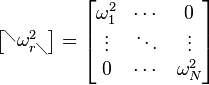$\begin{bmatrix} ^\diagdown \omega_{r\diagdown}^2 \end{bmatrix}=\begin{bmatrix} \omega_1^2 & \cdots & 0 \\ \vdots & \ddots & \vdots \\ 0 & \cdots & \omega_N^2 \end{bmatrix}$ and$\begin{bmatrix} \Psi \end{bmatrix}=\begin{bmatrix} \begin{Bmatrix} \psi_1 \end{Bmatrix} \begin{Bmatrix} \psi_2 \end{Bmatrix} \cdots \begin{Bmatrix} \psi_N \end{Bmatrix} \end{bmatrix}$

A simple example using our 2 DOF model can help illustrate the concepts. Let both masses have a mass of 1 kg and the stiffness of all three springs equal 1000 N/m. The mass and stiffness matrix for this problem are then: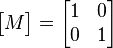$\begin{bmatrix}M\end{bmatrix}=\begin{bmatrix}1 & 0\\ 0 & 1\end{bmatrix}$ and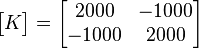$\begin{bmatrix}K\end{bmatrix}=\begin{bmatrix}2000 & -1000\\ -1000 & 2000\end{bmatrix}$

Then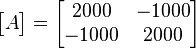$\begin{bmatrix}A\end{bmatrix}=\begin{bmatrix}2000 & -1000\\ -1000 & 2000\end{bmatrix}$.

The eigenvalues for this problem given by an eigenvalue routine will be: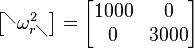$\begin{bmatrix} ^\diagdown \omega_{r\diagdown}^2 \end{bmatrix}=\begin{bmatrix} 1000 & 0 \\ 0 & 3000 \end{bmatrix}$

The natural frequencies in the units of hertz are then (remembering$\omega=2 \pi f$)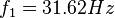$f_1=31.62 Hz$ and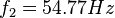$f_2=54.77 Hz$.

The two mode shapes for the respective natural frequencies are given as:$\begin{bmatrix} \Psi \end{bmatrix}=\begin{bmatrix} \begin{Bmatrix} \psi_1 \end{Bmatrix} \begin{Bmatrix} \psi_2 \end{Bmatrix} \end{bmatrix}= \begin{bmatrix} \begin{Bmatrix} -0.707 \\ -0.707 \end{Bmatrix}_1 \begin{Bmatrix} 0.707 \\ -0.707 \end{Bmatrix}_2 \end{bmatrix}$

Since the system is a 2 DOF system, there are two modes with their respective natural frequencies and shapes. The mode shape vectors are not the absolute motion, but just describe relative motion of the degrees of freedom. In this case, the first mode shape vector is saying that the masses are moving together in phase since they have the same value and sign. In the case of the second mode shape vector, each mass is moving in opposite direction at the same rate.

### Illustration of a multiple DOF problem

When there are many degrees of freedom, the best method of visualizing the mode shapes is by animating them. An example of animated mode shapes is shown in the figure below for a cantilevered I-beam. In this case, a finite element model was used to generate the mass and stiffness matrices and solve the eigenvalue problem. Even this relatively simple model has over a 100 degrees of freedom and hence as many natural frequencies and mode shapes. In general only the first few modes are important.

### Multiple DOF problem converted to a single DOF problem

The eigenvectors have very important properties called orthogonality properties. These properties can be used to greatly simplify the solution of multi-degree of freedom models. It can be shown that the eigenvectors have the following properties: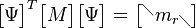$\begin{bmatrix}\Psi\end{bmatrix}^{T}\begin{bmatrix}M\end{bmatrix}\begin{bmatrix}\Psi\end{bmatrix}=\begin{bmatrix} ^\diagdown m_{r\diagdown} \end{bmatrix}$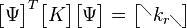$\begin{bmatrix}\Psi\end{bmatrix}^{T}\begin{bmatrix}K\end{bmatrix}\begin{bmatrix}\Psi\end{bmatrix}=\begin{bmatrix} ^\diagdown k_{r\diagdown} \end{bmatrix}$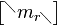$\begin{bmatrix} ^\diagdown m_{r\diagdown} \end{bmatrix}$ and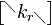$\begin{bmatrix} ^\diagdown k_{r\diagdown} \end{bmatrix}$ are diagonal matrices that contain the modal mass and stiffness values for each one of the modes. (Note: Since the eigenvectors (mode shapes) can be arbitrarily scaled, the orthogonality properties are often used to scale the eigenvectors so the modal mass value for each mode is equal to 1. The modal mass matrix is therefore an identity matrix)

These properties can be used to greatly simplify the solution of multi-degree of freedom models by making the following the coordinate transformation.$\begin{Bmatrix} x \end{Bmatrix}= \begin{bmatrix} \Psi \end{bmatrix} \begin{Bmatrix} q \end{Bmatrix}$

If we use this coordinate transformation in our original free vibration differential equation we get the following equation.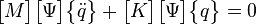$\begin{bmatrix}M\end{bmatrix}\begin{bmatrix} \Psi \end{bmatrix}\begin{Bmatrix}\ddot{q}\end{Bmatrix}+\begin{bmatrix}K\end{bmatrix} \begin{bmatrix} \Psi \end{bmatrix} \begin{Bmatrix} q\end{Bmatrix}=0$

We can take advantage of the orthogonality properties by premultiplying this equation by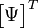$\begin{bmatrix}\Psi\end{bmatrix}^{T}$$\begin{bmatrix}\Psi\end{bmatrix}^{T}\begin{bmatrix}M\end{bmatrix}\begin{bmatrix} \Psi \end{bmatrix}\begin{Bmatrix}\ddot{q}\end{Bmatrix}+\begin{bmatrix}\Psi\end{bmatrix}^{T}\begin{bmatrix}K\end{bmatrix} \begin{bmatrix} \Psi \end{bmatrix} \begin{Bmatrix} q\end{Bmatrix}=0$

The orthogonality properties then simplify this equation to: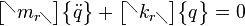$\begin{bmatrix} ^\diagdown m_{r\diagdown} \end{bmatrix} \begin{Bmatrix}\ddot{q}\end{Bmatrix}+\begin{bmatrix}^\diagdown k_{r\diagdown}\end{bmatrix} \begin{Bmatrix} q\end{Bmatrix}=0$

This equation is the foundation of vibration analysis for multiple degree of freedom systems. A similar type of result can be derived for damped systems. The key is that the modal and stiffness matrices are diagonal matrices and therefore we have "decoupled" the equations. In other words, we have transformed our problem from a large unwieldy multiple degree of freedom problem into many single degree of freedom problems that can be solved using the same methods outlined above.

Instead of solving for x, one is instead solving for q, referred to as the modal coordinates or modal participation factors.

It may be clearer to understand if$\begin{Bmatrix} x \end{Bmatrix}= \begin{bmatrix} \Psi \end{bmatrix} \begin{Bmatrix} q \end{Bmatrix}$ is written as: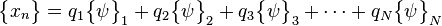$\begin{Bmatrix} x_n \end{Bmatrix}= q_1\begin{Bmatrix} \psi \end{Bmatrix}_1 +q_2\begin{Bmatrix} \psi \end{Bmatrix}_2 +q_3\begin{Bmatrix} \psi \end{Bmatrix}_3 +\cdots + q_N\begin{Bmatrix} \psi \end{Bmatrix}_N$

Written in this form we can see that the vibration at each of the degrees of freedom is just a linear sum of the mode shapes. Furthermore, how much each mode "participates" in the final vibration is defined by q, its modal participation factor.